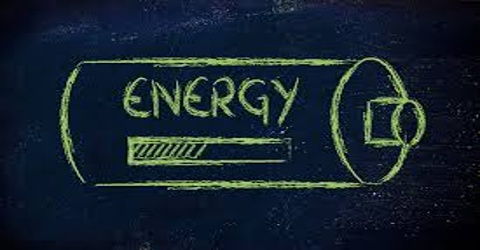# Unit and Dimension of Energy

Energy is the capability to do work. Energy comes in diverse forms: Heat (thermal), Light (radiant), Motion (kinetic) etc. Energy is defined as ability to do work.

Mathematically, Energy = Work.

Unit of energy: Since energy is measured with work, so unit of energy and work is the same. That means, unit of energy in S.I. system is Joule (J).

Dimension of energy: Dimension of energy and work is the same, [E] = [ML2T-2]

When a body is moving it gains kinetic energy. For example, a body of mass m moving with velocity r acquires kinetic energy of ½ mv2. Most common form of energy is the mechanical energy. Energy that remains in a body due to position and motion is called mechanical energy.

Mechanical energy is of two types; Kinetic energy and Potential energy.